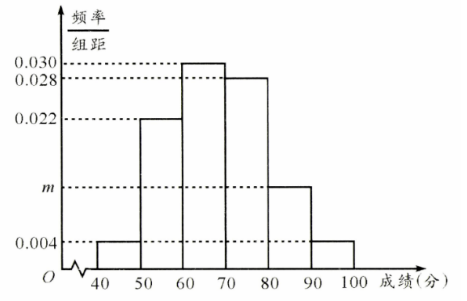(I) 求图中 $m$ 的值;
(II) 已知评分在 $[85,100]$ 的队伍有 4 支, 若从评分在 [80, 90) 的队伍中任选 两支队伍, 求这两支队伍至少有一支队 伍评分不低于 85 分的概率.
【答案】 解: ( I ) 由 $(0.004 \times 2+0.022+0.030+0.028+m) \times 10=1$, 解得 $m=0.012$.
(II) 由题意知不低于 90 分的队伍有 $50 \times 0.04=2$ 支, 故评分在 $[85,90)$ 的队伍有 2 支.

$\cdots \cdots 5$ 分